Ex 5.1

Chapter 5 Class 12 Continuity and Differentiability
Serial order wise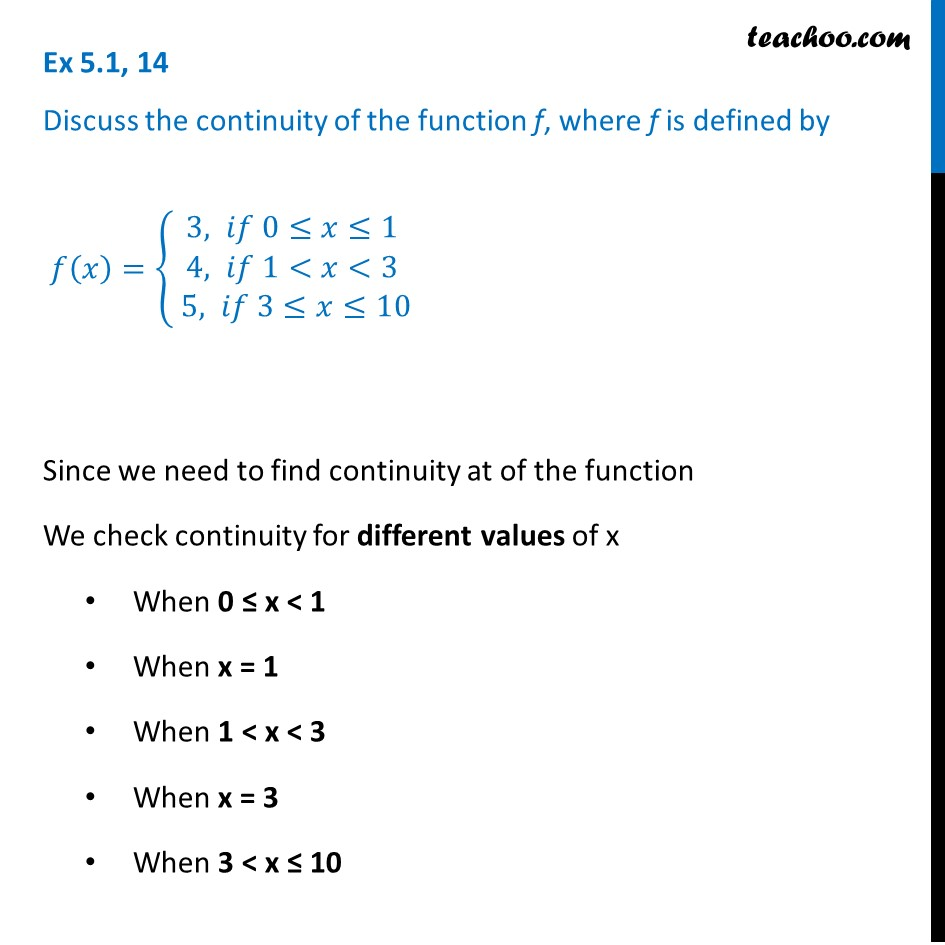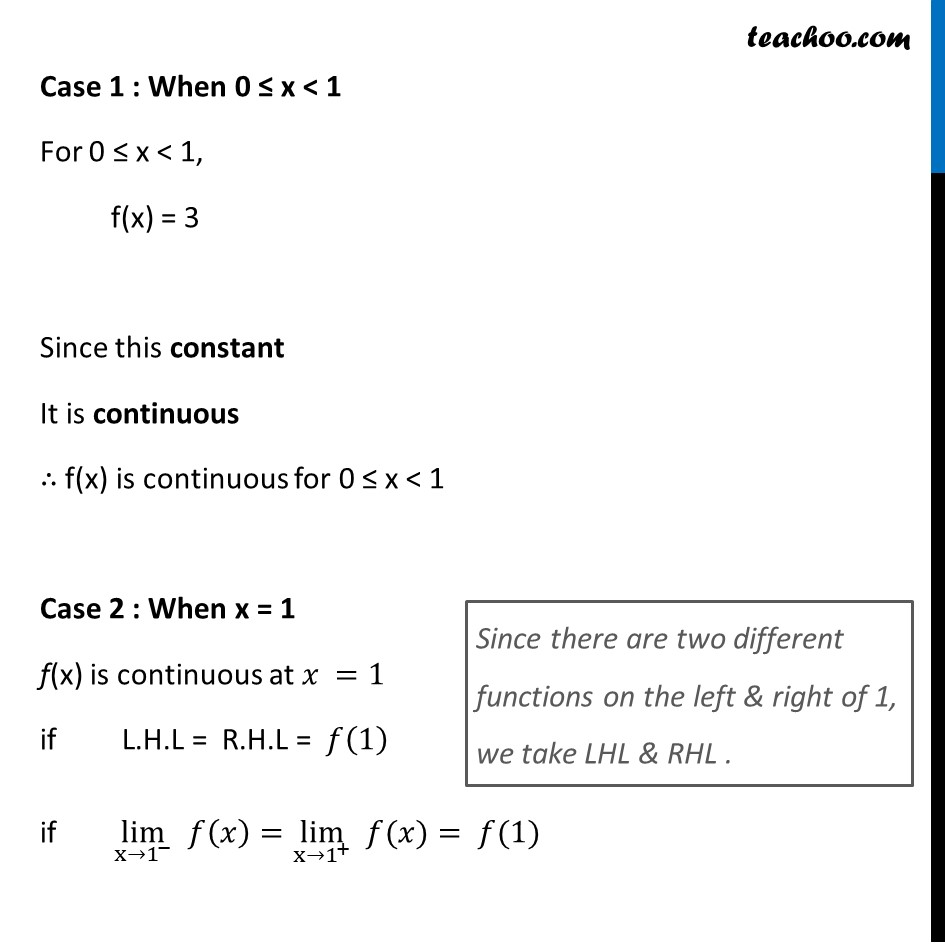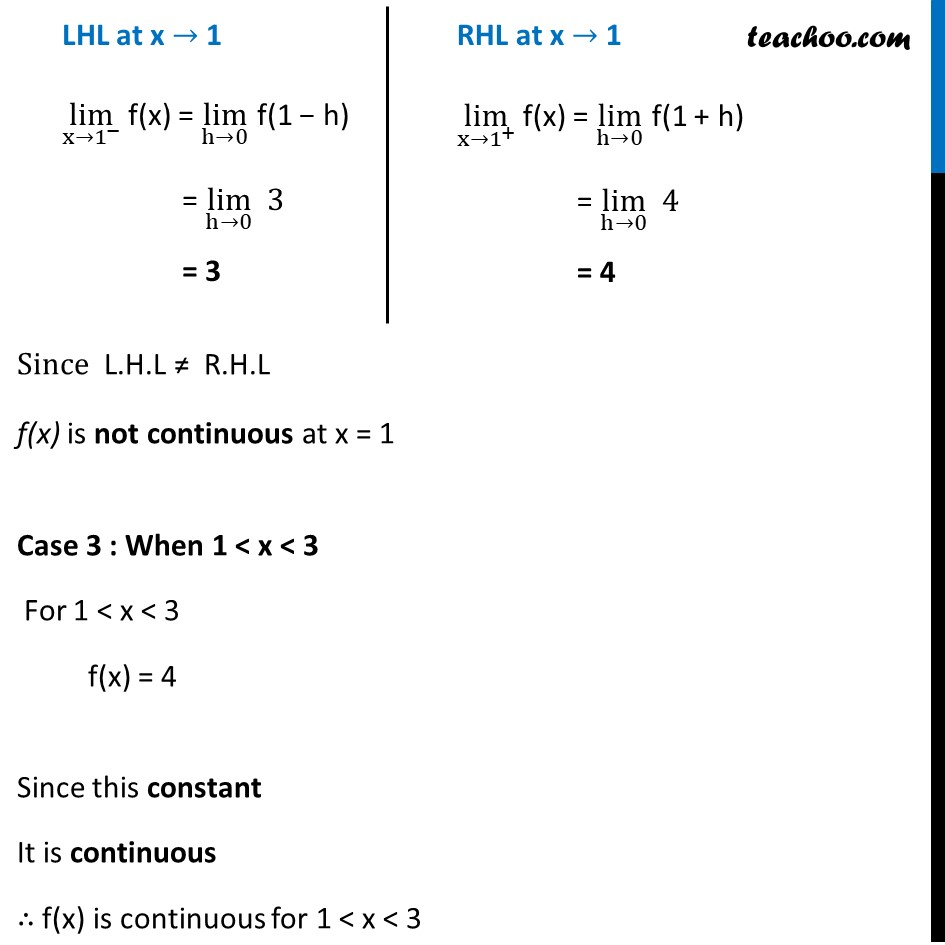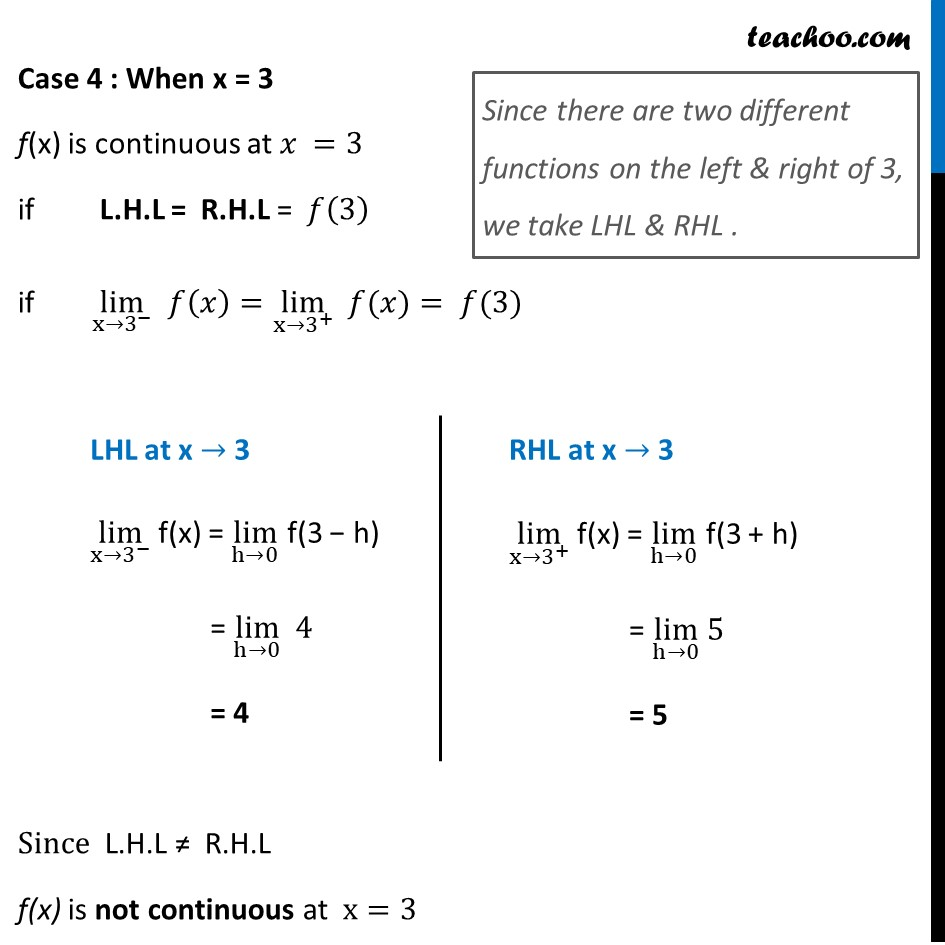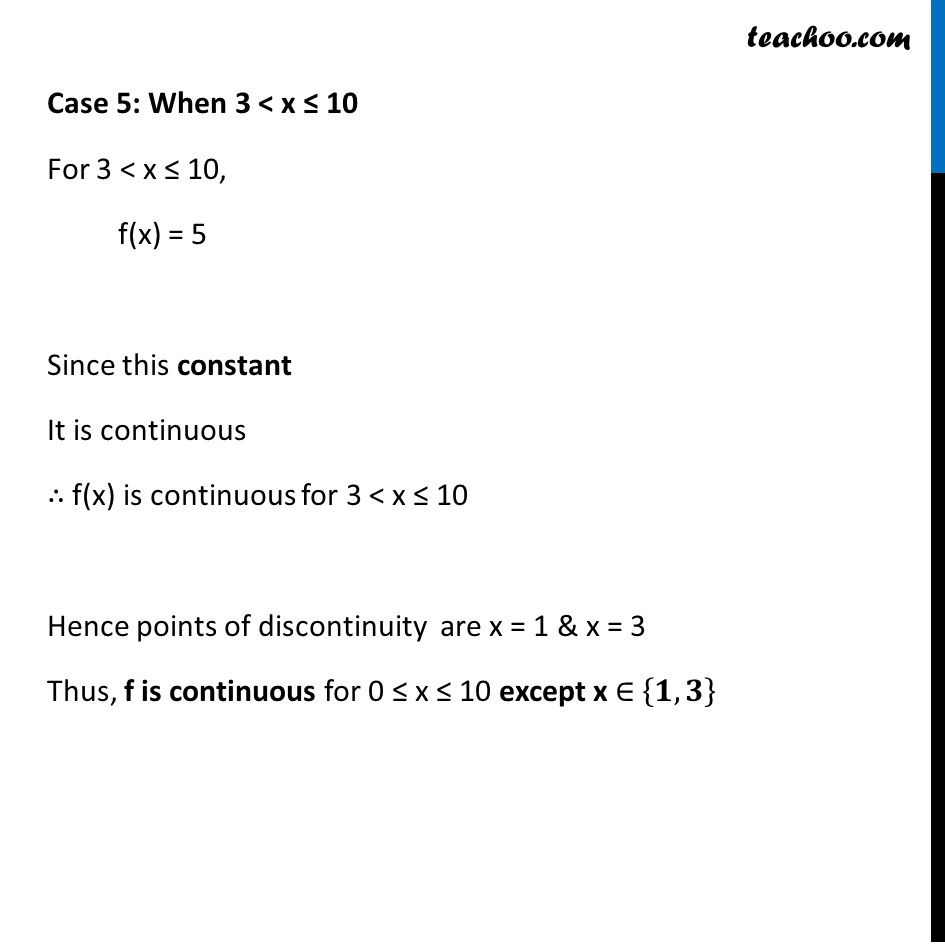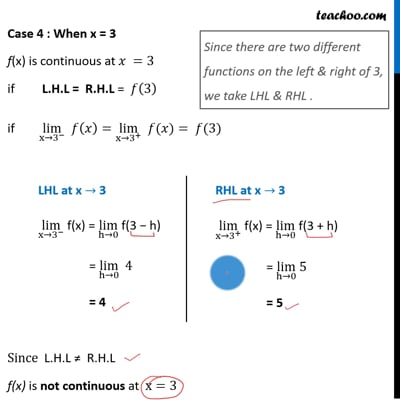This video is only available for Teachoo black users

Solve all your doubts with Teachoo Black (new monthly pack available now!)

### Transcript

Ex 5.1, 14 Discuss the continuity of the function f, where f is defined by 𝑓(𝑥)={█(3, 𝑖𝑓 0≤𝑥≤1@4, 𝑖𝑓 1<𝑥<3@ 5, 𝑖𝑓 3≤𝑥≤10)┤ Since we need to find continuity at of the function We check continuity for different values of x When 0 ≤ x < 1 When x = 1 When 1 < x < 3 When x = 3 When 3 < x ≤ 10 Case 1 : When 0 ≤ x < 1 For 0 ≤ x < 1, f(x) = 3 Since this constant It is continuous ∴ f(x) is continuous for 0 ≤ x < 1 Case 2 : When x = 1 f(x) is continuous at 𝑥 =1 if L.H.L = R.H.L = 𝑓(1) if lim┬(x→1^− ) 𝑓(𝑥)=lim┬(x→1^+ ) " " 𝑓(𝑥)= 𝑓(1) Since there are two different functions on the left & right of 1, we take LHL & RHL . LHL at x → 1 lim┬(x→1^− ) f(x) = lim┬(h→0) f(1 − h) = lim┬(h→0) 3 = 3 RHL at x → 1 lim┬(x→1^+ ) f(x) = lim┬(h→0) f(1 + h) = lim┬(h→0) 4 = 4 Since L.H.L ≠ R.H.L f(x) is not continuous at x = 1 Case 3 : When 1 < x < 3 For 1 < x < 3 f(x) = 4 Since this constant It is continuous ∴ f(x) is continuous for 1 < x < 3 Case 4 : When x = 3 f(x) is continuous at 𝑥 =3 if L.H.L = R.H.L = 𝑓(3) if lim┬(x→3^− ) 𝑓(𝑥)=lim┬(x→3^+ ) " " 𝑓(𝑥)= 𝑓(3) Since L.H.L ≠ R.H.L f(x) is not continuous at x=3 Since there are two different functions on the left & right of 3, we take LHL & RHL . LHL at x → 3 lim┬(x→3^− ) f(x) = lim┬(h→0) f(3 − h) = lim┬(h→0) 4 = 4 RHL at x → 3 lim┬(x→3^+ ) f(x) = lim┬(h→0) f(3 + h) = lim┬(h→0) 5 = 5 Case 4 : When x = 3 f(x) is continuous at 𝑥 =3 if L.H.L = R.H.L = 𝑓(3) if lim┬(x→3^− ) 𝑓(𝑥)=lim┬(x→3^+ ) " " 𝑓(𝑥)= 𝑓(3) Since L.H.L ≠ R.H.L f(x) is not continuous at x=3 Case 5: When 3 < x ≤ 10 For 3 < x ≤ 10, f(x) = 5 Since this constant It is continuous ∴ f(x) is continuous for 3 < x ≤ 10 Hence points of discontinuity are x = 1 & x = 3 Thus, f is continuous for 0 ≤ x ≤ 10 except x ∈ {𝟏,𝟑}